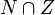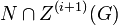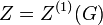# Nilpotent implies center is normality-large

This article gives the statement, and possibly proof, of the fact that in any nilpotent group, the subgroup obtained by applying a given subgroup-defining function (i.e., center) always satisfies a particular subgroup property (i.e., normality-large subgroup)
View all such subgroup property satisfactions OR View more information on subgroup-defining functions in nilpotent groups
This article gives the statement and possibly, proof, of an implication relation between two group properties. That is, it states that every group satisfying the first group property (i.e., nilpotent group) must also satisfy the second group property (i.e., group whose center is normality-large)
View all group property implications | View all group property non-implications
Get more facts about nilpotent group|Get more facts about group whose center is normality-large

## Statement

### Verbal statement

In a nilpotent group, the center is a normality-large subgroup; in other words, the intersection of the center with any nontrivial normal subgroup is a nontrivial normal subgroup.

## Proof

Given: A nilpotent group$G$ with center$Z$. A nontrivial normal subgroup$N$ of$G$.

To prove:$N \cap Z$ is nontrivial.

Proof:

Step no. Assertion/construction Facts used Given data used Previous steps used Explanation
1 Denote by$Z^{(i)}(G)$ the$i^{th}$ member of the upper central series of$G$. So$Z^{(0)}(G)$ is trivial,$Z^{(1)}(G) = Z$ is the center, and$Z^{(c)}(G) = G$ where$c$ is the nilpotency class of$G$.$G$ is nilpotent.
2 There exists some$i \ge 0$ such that$N \cap Z^{(i)}(G)$ is trivial and$N \cap Z^{(i + 1)}(G)$ is nontrivial.$N$ is nontrivial. Step (1) [SHOW MORE]
3$[G,Z^{(i + 1)}(G)] \le Z^{(i)}(G)$. Definition of upper central series
4$[G,N] \le N$.$N$ is normal in$G$.
5$[G,N \cap Z^{i+1}(G)] \le [G,N] \cap [G,Z^{(i+1)}(G)$. By definition of commutator of two subgroups in terms of the commutators being a generating set.
6$[G,N \cap Z^{(i+1)}(G)]$ is trivial. Steps (2),(3),(4),(5) [SHOW MORE]
7$i = 1$, and$N \cap Z^{(1)}(G) = N \cap Z$ is nontrivial. Steps (2), (6) <toggledisplay>By Step (6),$N \cap Z^{(i+1)}(G)$ is contained in$Z = Z^{(1)}(G)$, so we have a nontrivial subgroup contained in both$N$ and$Z$. So,$N \cap Z$ is nontrivial. Reviewing the definition of$i$ from Step (2), we obtain that$i$ must equal 1.